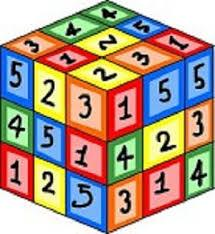# Multiply 3766

Peter had a number in mind and said: When I divide this unknown number by the square root of 27 and then multiply by three, I get an unknown number. What number did Peter think?

x =  0

### Step-by-step explanation:Did you find an error or inaccuracy? Feel free to write us. Thank you!

Tips for related online calculators
Do you have a linear equation or system of equations and looking for its solution? Or do you have a quadratic equation?